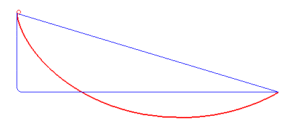# Quickest Way DownCan you predict which path will be the quickest? Test your prediction. Why do you think this path is the quickest? What happens if you release the ball at a different point?
Maths

The shortest distance between two points is a straight line, but thanks to gravity and acceleration, it is not the quickest way down.
Instead, you will discover that the quickest way down is one of the curves.The curve is called a brachistochrone, which means “shortest time”. This curve is quicker because it starts off steeply which allows the ball to accelerate quickly.
The other curve is related. This curve will take the same time no matter where the ball is released.This curve is called a tautochrone, which means “same time”.
The two curves are related because they are both part of the curve drawn by a point on a circle as it rolls along the ground. This curve is called a cycloid.

A tautochrone can start at any point on the cycloid, but always ends horizontally. A brachistochrone can end at any point on the cycloid, but always starts at the top of the cusp.

History

In 1696, famous Swiss mathematician, Johann Bernoulli set a challenge for other mathematicians of the time. The challenge was to find the curve that would give the quickest way down. Along with Johann Bernoulli, five other mathematicians submitted answers, they were Jakob Bernoulli (Johann’s brother), Isaac Newton, Gottfried Leibniz, Ehrenfried Walther von Tschirnhaus and Guillaume de l’Hôpital.

The mathematicians all solved the problems in different ways, but all got the same answer, with some noting that the curve was part of a cycloid, and related to the tautochrone that had been discovered 40 years earlier.

This problem led to new mathematical tools for solving problems, which are still used today to solve problems involving the quickest time, or shortest distance.

PeopleJohann Bernoulli 1667 – 1748 Bernoulli was a Swiss mathematician, famous for his work on the mathematics of change, known as calculus. Bernoulli had a jealous and competitive relationship with his brother Jacob Bernoulli, who was also a mathematician. When Johann set a challenge to find the quickest way down, his solution contained a mistake, and so he tried to pass his brother’s solution as his own. Isaac Newton 1642 – 1726 Newton is one of the greatest mathematicians and scientists of all time. Newton is famous for his laws of motion and gravitation, as well as his work on optics and fluids. Newton developed the mathematics of change, known as calculus, which he used to study the orbits of the planets. Newton entered Bernoulli’s challenge to find the quickest way down. The problem took Bernoulli two weeks to solve, but Newton was able to solve the problem in just one nightApplications

You will find the brachistochrone curve in rollercoasters, ski jumps and surfing. These are all examples of wanting to descend as fast as possible.

Maths at Home

The curve used in this activity is called a cycloid. A cycloid is the curve drawn by a point of the rim of a wheel as it rolls along the ground. If we roll a circle inside another circle we get spirals. These are the same kinds of patterns made by a Spirograph. Try this online Spirograph (called Inspirograph) and see what kind of patterns you can make,

Can you make a cycloid? What do you need to make a cycloid?